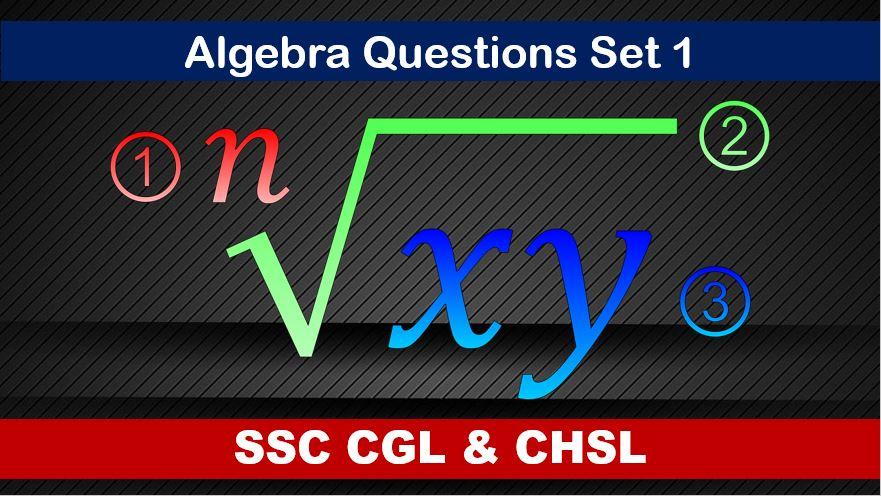Monday, September 27, 2021
Home > algebra > SSC Algebra Questions and Solutions Set 1

# SSC Algebra Questions and Solutions Set 1SSC CGL CHSL Algebra
Algebra Questions Sets
Set 1Set 2Set 3Set 4Set 5
Set 6

SSC Algebra Questions and Solutions Set 1 : Ques No 1

If x + 1/x = 2, then the value of x5 + 1/x5 is

Options:

A. 2

B. 3

C. 4

D. 5

Solution:

SSC Algebra Questions and Solutions Set 1 : Ques No 2

If x + a/x = b, then the value of (x2 + bx + a) / (bx2 – x3) is

Options:

A. b/a

B. ab

C. a + b

D. 2b / a

Solution:

SSC Algebra Questions and Solutions Set 1 : Ques No 3

If a2 + 1/a2 = 98 (a > 0), then the value of a3 + 1/a3 is

Options:

A. 535

B. 1030

C. 790

D. 970

Solution:

SSC Algebra Questions and Solutions Set 1 : Ques No 4

The value of (x + 1/x)(x – 1/x)(x2 + 1/x2 – 1)(x2 + 1/x2 + 1) is

Options:

A. x6 + 1/x6

B. x8 + 1/x8

C. x8 – 1/x8

D. x6 – 1/x6

Solution:

SSC Algebra Questions and Solutions Set 1 : Ques No 5

If x + 2/x = 3, then the value of (x2 + x + 2) / (x2(3 – x)) is

Options:

A. 0

B. 1

C. 2

D. 3

Solution:

SSC Algebra Questions and Solutions Set 1 : Ques No 6

If x = ay, y = bx, then the value of 1/(1 + a) + 1/(1 + b) is

Options:

A. 0

B. 1

C. -1

D. 2

Solution:

SSC Algebra Questions and Solutions Set 1 : Ques No 7

If a = 99, then the value of [a(a2 + 3a + 3)] is

Options:

A. 99999

B. 1000000

C. 999999

D. 9999999

Solution:

SSC Algebra Questions and Solutions Set 1 : Ques No 8

If 2a + 3b = 4, then the value of 8a3 + 27b3 + 72ab is

Options:

A. 54

B. 48

C. 72

D. 64

Solution:

SSC Algebra Questions and Solutions Set 1 : Ques No 9

If a + b = 1 and a3 + b3 + 3ab = k, then the value of k is

Options:

A. 1

B. 3

C. 5

D. 7

Solution:

SSC Algebra Questions and Solutions Set 1 : Ques No 10

If 3x + 2y = 12 and xy = 6, then the value of 9x2 + 4y2 is

Options:

A. 72

B. 74

C. 76

D. 80

SSC Algebra Questions and Solutions Set 1 : Ques No 11

In an objective examination of 90 questions, 5 marks are allotted for every correct answer and 2 marks are deducted for every wrong answer. After attempting all the 90 questions a students got a total of 387 marks. Find the number of questions that he attempted wrong

Options:

A. 9

B. 10

C. 11

D. 12

SSC Algebra Questions and Solutions Set 1 : Ques No 12

The ages of Shivali and Tanisha are in the ratio of 11:7 respectively. After 8 years the ratio of their ages will be 15:11. What is the difference in years between their ages?

Options:

A. 4 years

B. 6 years

C. 8 years

D. 10 years

SSC Algebra Questions and Solutions Set 1 : Ques No 13

On a scale that measures the intensity of a certain phenomenon, a reading of (n+1) corresponds to an intensity that is 10 times the intensity corresponding to a reading of n. On that scale, the intensity corresponding to a reading of 8 is how many times as great as the intensity corresponding to a reading of 3?

Options:

A. 500

B. 35

C. 105

D. 50

SSC Algebra Questions and Solutions Set 1 : Ques No 13

Naveen purchased two oranges, 3 apples and 5 bananas and paid Rs 40. Had Naveen purchased 3 oranges, 5 apples and 9 bananas, He would have to pay Rs 64. Gagan demanded only 1 orange, 1 apple and 1 banana. If Naveen purchased only what was demanded by Gagan, then how much would he have paid (in Rs)?

Options:

A. 16

B. 28

C. 36

D. 24